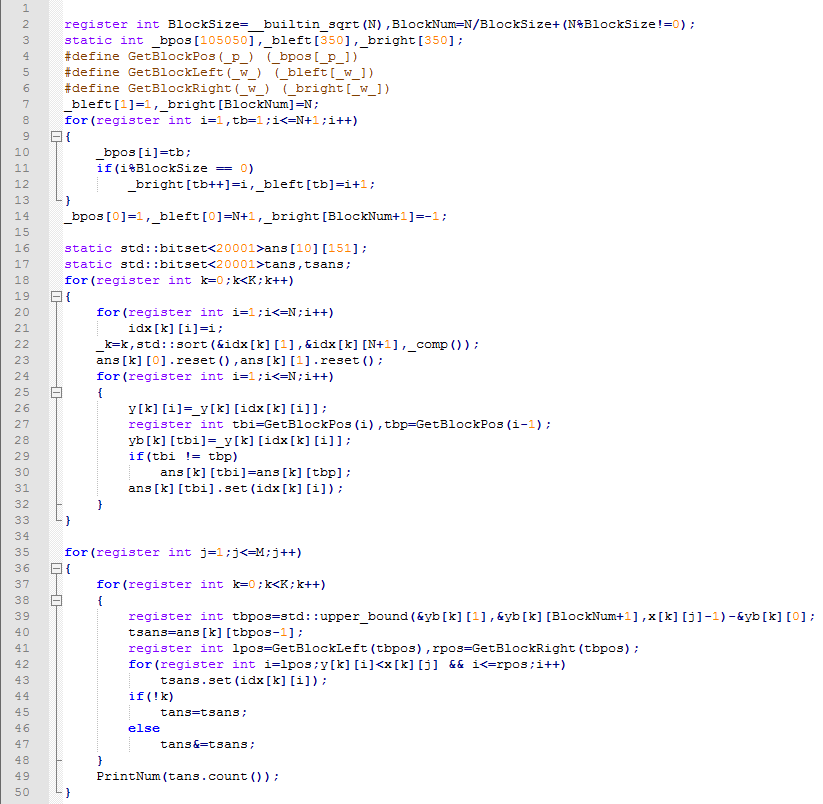# [研学] 偏序问题的研究

• ### 高维偏序…

# [BJOI2018] 链上二次求和

• ### 题目描述

YJC 有一棵 $$n$$ 个节点的树， $$i$$、$$i+1$$ （$$1 \leq i < n$$）节点间有一条边，第 $$i$$ 个点的权值为整数 $$a_i$$ 。

# YZOJ P4611 区间加多项式（YJC 的数组/多项式？）

• ### 题目描述

YJC 在 AK 了一场校内之后，对其中的一题（P2036 「A Simple Data Structure Problem II 」）产生了兴趣。

$$opt=1$$，给定 $$L, R$$，输出 $$\sum\limits_{i=L}^R c_i$$ 的值对 $$998244353$$ 取模后的结果；

$$opt=0$$，给定 $$L, R$$ 以及 $$K$$ 次多项式 $$f(x)=\sum\limits_{k=0}^K a_kx^k$$，对 $$c_L, c_{L+1}, \cdots, c_R$$ 分别加上 $$f(1), f(2), \cdots, f(R-L+1)$$ 的值。

# YZOJ P4637 [CSP-S 2019 五校联训 Round 2]由比滨结衣(sqrt)

• ### 题目描述

1 l r x 将区间 $$[l,r]$$ 中的所有数变为 $$x$$。

2 l r x 查询区间 $$[l,r]$$ 中数字 $$x$$ 的出现次数。

• ### 题目描述

1、询问点 $$x$$ 和点 $$y$$ 之间的路径上的所有点（包括点 $$x$$ 和点 $$y$$ ）的点权是否构成一个从 $$1$$ 开始的排列。

2、将 $$A_x$$ 修改为 $$y$$。

# YZOJ P4444 [APIO2019]路灯

• ### 题目描述

$$toggle i$$：切换第 $$i$$ 个路灯的状态。具体地说，若路灯原来亮起，则现在将熄灭；若路灯原来熄灭，则现在将亮起。

$$query a b$$：自动驾驶的士部门的负责人想知道，从 $$0$$ 时刻起到当前时刻，有多少个时刻满足：自动驾驶的士能够从站点 $$a$$ 出发到达站点 $$b$$ 。

• ### 输入格式

$$toggle i$$ $$(1 \leq i \leq n)$$：该时刻切换了第 $$i$$ 个路灯的状态。

$$query a b$$ $$(1 \leq a < b \leq n+1)$$：计算从 $$0$$ 时刻起到该时刻，共有多少个时刻满足的士能从站点 $$a$$ 出发到达站点 $$b$$ 。

…

# YZOJ P2050 [FJOI2013]圆形游戏

• ### 题目描述

1，选定一个圆 $$A$$，把 $$A$$ 以及所有完全在 $$A$$ 内部的圆都删除；

2，如果在自己回合无法找到可删除的圆，则输掉比赛。

• ### 数据规模与约定

$$100\%$$ 的数据满足 $$T \leq 100$$，$$n \leq m20000$$，$$\left|x\right|, \left|y\right|, r \leq 10^8$$ 。

…

# YZOJ P3942 gss2加强版

• ### 题目描述

U x y：将第 $$x$$ 个数修改成 $$y$$ ；

Q x y：计算从第 $$x$$ 个数至第 $$y$$ 个数中不同数的和并输出。如对于一段数 $$1,2,3,2,7$$，它的值是 $$13=1+2+3+7$$ 。

• ### 数据规模与约定

$$n \leq 100000 , m \leq 100000$$

Source： BZOJ 2883…

# YZOJ P2905 [PA2014]Druzyny

• ### 题目描述

OI组的神犇们决定把这题改造一下：

dick32165401：改成只有编号连续的的一段才可以分一组。

runzhe2000：判定可行解可能会被爆搜水过，最大化分的组数就不那么容易水过了。

E.Space：不仅要最大化组数，还要求出最大化组数的方案数。

ct：数据范围就出100万好了。

• ### 样例输出

Source: BZOJ 3711